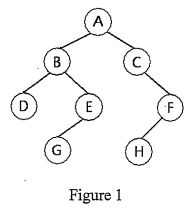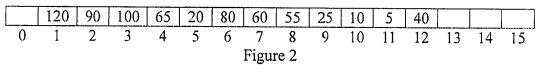lvy>試卷(2022/08/12)

# 106 年 - 106 國立中山大學_碩士班招生考試_電機系(丙組)：資料結構#110221

【非選題】
1.
1. Suppose we have a byte-addressable machine, i.e., cach byte is accessed via an address. Let the locations of an array be allocated in a row-major manner. In a program segment, we declare an PPby
int PP[20,30]；
Let each integer take 4 bytes and the location of this array start with address 1000. Please answer the following questions:

【題組】 1.1  How many integers can be stored in the array PP?

【非選題】
2.【題組】1.2  How many bytes does the array PP itself take?

【非選題】
3.【題組】1.3  What is the starting address of the element PP[10,5]?

【非選題】
4.【題組】1.4  What is the starting address of the element PP[5, 10]?

【非選題】
5.【題組】1.5  What is the starting address of the last element in PP?

【非選題】
6.
2. Suppose we use a singly linked list to store integers in its nodes. Assume that, initially, 100 is stored in the first node starting at address 2000, 200 is stored in the second node starting at address 500, 300 is stored in the third node starting at address 1000, and 400 is stored in the fourth node starting at address 100. Let the starting address of the first node of the list is stored in the variable named head. Let an integer take 4 bytes and a pointer (or address) take 8 bytes. Please answer the following questions:

【題組】 2.1  What is the size, in the number of bytes, of each node of the list?

【非選題】
7.【題組】2.2  What is stored in the variable head?

【非選題】
8.【題組】2.3  What is the content of the first node of the list?

【非選題】
9.【題組】2.4  What is the content of the last node of the list?

【非選題】
10.【題組】2.5  Suppose we delete the second node from the list. After deletion, what is the content of the first node of the resulting list?

【非選題】
11.

3. Usually, there are three ways to display the nodes of a binary tree: preorder, inorder, and postorder. Consider Figure 1 and answer the following questions:【題組】 3.1  What is the fifth node of the preorder sequence of this tree?

【非選題】
12.【題組】3.2  What is the fifth node of the inorder sequence of this tree?

【非選題】
13.【題組】3.3  What is the fifth node of the postorder sequence of this tree?

【非選題】
14.

4. Initially, the array AA shown in Figure 2 stores a max heap with 12 integers. Note that A i not used. Suppose we Insert 105 into the heap and then make it into a max heap.【題組】 4.1  What is the content of A of the resulting array?

【非選題】
15.【題組】4.2  What is the content of A of the resulting array?

【非選題】
16.【題組】4.3  What is the content of A of the resulting array?

【非選題】
17.【題組】4.4  What is the content of A of the resulting array?

【非選題】
18.【題組】4.5  We delete the root node from the heap and make it into a max heap again. What is the content of the A of the resulting array?

【非選題】
19.
5. Let one-node binary tree have a depth of 1. Suppose we have a complete binary tree T of depth 100.

【題組】 5.1  What is the minimum number of nodes in T?

【非選題】
20.【題組】5.2  What is the maximum number of nodes in T?

【非選題】
21.【題組】5.3  There are n possible complete binary trees of depth 100. What is n?

【非選題】
22.【題組】5.4  Suppose we number the nodes T by starting with the node on level 1, and continuing with the nodes on level 2, and so on. Nodes on any level are numbered from left to right. Let the first node be numbered 1, the second node be numbered 2, and so on. For a node numbered 200, what is its left node numbered?

【非選題】
23.【題組】5.5  Following above, for a node numbered 3001, what is its parent node numbered?

【非選題】
24.
6. Suppose we have the following 11 integers:
44,30,95,33,50,82,18,55,70, 64,26
Please create a hash table AA with 13 entries declared by
int AA；
Let's insert the integers one by one and from the left to the right into the hash table. Assume the hash function is h(k) = k%13 and linear probing is used for collision resolution. Note that % yields the remainder when one integer is divided by another, for example, 20%13 = 7 and 5%13 = 5. Please answer the following questions:

【題組】 6.1  What is the content of A[O]?

【非選題】
25.【題組】6.2  What is the content of A?

【非選題】
26.【題組】6.3  What is the content of A?

【非選題】
27.【題組】6.4  What is the content of A?

【非選題】
28.【題組】6.5  What is the content of A?

【非選題】
29.
7. Suppose we have 12 integers: 60, 40, 25, 10, 50, 80, 15, 65, 30, 90, 20, 55. Construct a binary search tree by inserting the integers, starting from 60, one after another. Please answer the following questions for the binary search tree:

【題組】 7.1  What is the left child of the root node?

【非選題】
30.【題組】7.2  What is the right child of the node containing 80?

【非選題】
31.【題組】7.3  What is the left child of the node containing 25?

【非選題】
32.【題組】7.4  What is the right child of the node containing 55?

【非選題】
33.【題組】7.5  What is the height of the binary tree? Let one-node tree have a depth of 1.

### 懸賞詳解

#### 國二數學下第二次

3. ( ) 如右圖，已知 ，依下列敘述，利用尺規作圖完成圖形。 【作法】1 分別以 B、C 兩點為圓心，大於  長為半徑畫弧，設兩弧交於 A 點。 2 連接 ，得�...